Processing ......FreeComputerBooks.com Links to Free Computer, Mathematics, Technical Books all over the World

Mathematical Logic - Computability, Set Theory, Model Theory, Proof Theory, etc.
Related Book Categories:
 Theory of Computation and Computing Semantics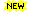LISP and Common LISP Computational Complexity Calculus and Mathematical Analysis Computational and Algorithmic Mathematics
•A Friendly Introduction to Mathematical Logic (Chris Leary)

In this user-friendly book, readers with no previous study in the field are introduced to the basics of model theory, proof theory, and computability theory, leading to rigorous proofs of Gödel's First and Second Incompleteness Theorems.

•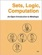Sets, Logic, Computation: An Open Introduction to Metalogic

It covers naive set theory, first-order logic, sequent calculus and natural deduction, the completeness, compactness, and Löwenheim-Skolem theorems, Turing machines, and the undecidability of the halting problem and of first-order logic.

•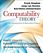Computability Theory: Introduction to Recursion Theory

Computability Theory originated with the seminal work of Gödel, Church, Turing, Kleene and Post in the 1930s. These texts provide concise, comprehensive, and authoritative introduction to contemporary computability theory, techniques, and results.

•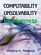Computability, Unsolvability, Randomness (Stephen G. Simpson)

The author exposits Turing's 1936 theory of computability and unsolvability, as subsequently developed by Kleene and Post. This theory is of the essence in theoretical computer science and in the study of unsolvable mathematical problems.

•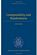Computability and Randomness (Andre Nies)

The complexity and the randomness aspect of a set of natural numbers are closely related. This book includes a detailed treatment of Turing's theory of computability and unsolvability as subsequently developed by Kleene, Post, Friedberg, etc.

•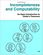Incompleteness and Computability: Gödel's Theorems

This book is an introduction to metamathematics and Gödel's Theorems. It covers recursive function theory, arithmetization of syntax, the first and second incompleteness theorem, models of arithmetic, second-order logic, and the lambda calculus.

•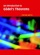An Introduction to Gödel’s Theorems (Peter Smith)

The formal explanations are interwoven with discussions of the wider significance of the two Gödel's Theorems. It will be of equal interest to mathematics students taking a first course in mathematical logic.

•What is Mathematics: Godel's Theorem and Around (K. Podnieks)

This accessible book gives a new, detailed and elementary explanation of the Gödel's Incompleteness Theorems and presents the Chaitin results and their relation to the da Costa-Doria results, which are given in full, but with no technicalities.

•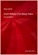Gödel Without (Too Many) Tears (Peter Smith)

How is this remarkable result of Gödel's Theorems established? This short book explains. The aim is to make the Theorems available, clearly and accessibly, even to those with a quite limited formal background.

•Introduction to Mathematical Logic (Vilnis Detlovs, et al)

This book explores the principal topics of mathematical logic. It covers propositional logic, first-order logic, first-order number theory, axiomatic set theory, and the theory of computability. Discusses the major results of Gödel, Church, Kleene, Rosser, and Turing.

•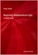Beginning Mathematical Logic: A Study Guide (Peter Smith)

It introduces the core topics and recommends the best books for studying these topics enjoyably and effectively. An invaluable resource both for those wanting to teach themselves new areas of logic and for those looking for supplementary reading.

•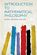Introduction to Mathematical Philosophy (Bertrand Russell)

Requiring neither prior knowledge of mathematics nor aptitude for mathematical symbolism, the book serves as essential reading for anyone interested in the intersection of mathematics and logic and in the development of analytic philosophy.

•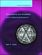Computability and Complexity: From a Programming Perspective

This book is an introduction to the basic concepts of computability, complex, and the theory of programming languages. Its goal is to build a bridge between computability and complexity theory and other areas of computer science, especially programming.

•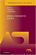Model-Theoretic Logics (Jon Barwise, et al)

This book brings together several directions of work in model theory between the late 1950s and early 1980s. It provides an introduction to the subject as a whole, as well as to the basic theory and examples. Many chapters can be read independently.

•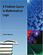A Problem Course in Mathematical Logic (Stefan Bilaniuk)

It is intended to serve as the text for an introduction to mathematical logic for undergraduates with some mathematical sophistication. It supplies definitions, statements of results, and problems, along with some explanations, examples, and hints.

•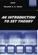An Introduction to Set Theory (William A. R. Weiss)

This book covers the basics: relations, functions, orderings, finite, countable, and uncountable sets, and cardinal and ordinal numbers, gives students sufficient grounding in a rigorous approach to the revolutionary results of set theory.

•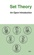Set Theory: An Open Introduction (Richard Zach)

This book is a brief introduction to the philosophy of set theory. It is written for students with a little background in logic, and some high school mathematics, aims to scratch the tip of the surface of the philosophy of set theory.

•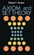Axioms and Set Theory (Robert Andre)

This book provides a very clear and well-developed approach. It covers axioms and set theory for the mathematics student on the upper undergraduate or graduate level. Throughout, emphasis is on axioms and theorems; proofs are informal.

•Proof, Sets, and Logic (M. Randall Holmes)

Addressing the importance of constructing and understanding mathematical proofs, this book introduces key concepts from logic and set theory as well as the fundamental definitions of algebra to prepare readers for further study in the field of mathematics.

•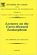Lectures on the Curry-Howard Isomorphism (M. H. Sorensen, et al)

This book gives an introduction to parts of proof theory and related aspects of type theory relevant for the Curry-Howard isomorphism. It can serve as an introduction to any or both of typed lambda-calculus and intuitionistic logic.

•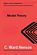Model Theory (C. Ward Henson)

It is an up-to-date textbook of model theory taking the reader from first definitions to Morley's theorem and the elementary parts of stability theory, introduces the model theory of first-order logic, avoiding syntactical issues not too relevant to model theory.

•Model Theory, Algebra, and Geometry (Deirdre Haskell, et al)

Tis book gives the necessary background for understanding both the model theory and the mathematics behind the apps, begins with an introduction to model theory, broadens into three components: pure model theory, geometry, and the model theory of fields.

•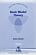Basic Model Theory (Kees Doets)

As the title indicates, this book introduces the reader to what is basic in model theory. A special feature is its use of the Ehrenfeucht game by which the reader is familiarised with the world of models.

•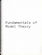Fundamentals of Model Theory (William Weiss, et al)

This book is a concluding discussion focuses on the relationship between proofs and formal derivations, and the role proofs may play as part of a general theory of evidence. It is a primer which will give someone a self contained overview of the subject.

•Euclid and His Twentieth Century Rivals (Nathaniel Miller)

Twentieth-century developments in logic and mathematics have led many people to view Euclid's proofs as inherently informal, especially due to the use of diagrams in proofs. It introduces a diagrammatic computer proof system, based on this formal system.

•Elementary Calculus: An Infinitesimal Approach (H. Jerome Keisler)

This first-year calculus book is centered around the use of infinitesimals. It is concerned with the infinitesimal approach originally set forth by Newton and Leibnitz, using Non-standard analysis.

•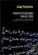Nonstandard Analysish (Jaap Ponstein, et al.)

This book is concerned with an attempt to introduce the infinitesimals and the other 'nonstandard' numbers in a naive, simpleminded way. Nevertheless, the resulting theory is hoped to be mathematically sound, and to be complete within obvious limits.

•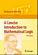A Concise Introduction to Mathematical Logic (W. Rautenberg)

This is a well-written introduction to the beautiful and coherent subject of mathematical logic. It contains classical material such as logical calculi, beginnings of model theory, and Goedel's incompleteness theorems, as well as some topics motivated by applications.

•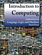Introduction to Computing: Language, Logic, and Machines

This book focuses on how to describe information processes by defining procedures, how to analyze the costs required to carry out a procedure, and the fundamental limits of what can and cannot be computed mechanically.

•Language, Proof and Logic (Jon Barwise, et al)

This book covers first-order language in a method appropriate for first and second courses in logic, and is specially useful to undergraduates of philosophy, computer science, mathematics, and linguistics.

•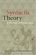Syntactic Theory: A Formal Introduction (Ivan A. Sag, et al)

This is a textbook that makes it truly fun to teach introductory syntax. It is thoroghly data-driven and teaches the student to pay attention to empirical details and to find linguistic patterns and explanations for them.

•Mathematics in the Age of the Turing Machine (Thomas C. Hales)

Computers have rapidly become so pervasive in mathematics that future generations may look back to this day as a golden dawn. The article gives a survey of mathematical proofs that rely on computer calculations and formal proofs.

•Set Theoretic Approach to Algebraic Structures in Mathematics

This book brings out how sets in algebraic structures can be used to construct the most generalized algebraic structures, like set linear algebra / vector space, set ideals in groups and rings and semigroups, and topological set vector spaces.

•Automata Theory: An Algorithmic Approach (Javier Esparza, et al)

This textbook presents Automata Theory from a fresh viewpoint inspired by its main modern application, program verification, where automata are viewed as data structures for the algorithmic manipulation of sets and relations.

•Logic and Automata: History and Perspectives (Jorg Flum, et al)

This book gives a consolidated overview of the research results achieved in the theory of automata and logic, covers many different facets of logic and automata theory, emphasizing the connections to other disciplines such as games, algorithms, etc.

•Logic and the Foundations of Game and Decision Theory

This book collects together revised papersoriginally presented at the 7th Conference onLogic and the Foundations of Game and DecisionTheory (LOFT). LOFT is a key venue forpresenting research at the intersection of logic,economics and computer science.

•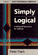Simply Logical: Intelligent Reasoning by Example (Peter Flach)

This book is an introduction to Prolog programming for artificial intelligence covering both basic and advanced AI material. A unique advantage to this work is the combination of AI, Prolog and Logic.

•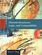Discrete Structures, Logic, and Computability (James L. Hein)

This book introduces beginning computer science and computer engineering students to the fundamental techniques and ideas used by computer scientists today, focusing on topics from the fields of mathematics, logic, and computer science itself.

•Logic for Computer Science: Automatic Theorem Proving

This book introduces mathematical logic with an emphasis on proof theory and procedures for algorithmic construction of formal proofs. It is useful for the formalization of proofs and basics of automatic theorem proving.

•The Haskell Road to Logic, Maths and Programming (Kees Doets)

The purpose of this book is to teach logic and mathematical reasoning in practice, and to connect logical reasoning with computer programming. Haskell is based on a logical theory of computable functions called the lambda calculus.

•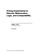Prolog Experiments in Discrete Math, Logic, and Computability

This book contains programming experiments that are designed to reinforce the learning of discrete mathematics, logic, and computability. The Prolog programming language is the tool used for the experiments in this book.

•Let Over Lambda - 50 Years of Lisp (Doug Hoyte)

This book is one of the most hardcore computer programming books out there. Starting with the fundamentals, it describes the most advanced features of the most advanced language: Common Lisp.

•Higher Topos Theory (Jacob Lurie)

This book presents the foundations of Higher Topos Theory, using the language of weak Kan complexes, and shows how existing theorems in algebraic topology can be reformulated and generalized in the theory's new language.

•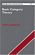Basic Category Theory (Tom Leinster)

Assuming little mathematical background, this short introduction to Category Theory is ideal for beginning graduate students or advanced undergraduates learning category theory for the first time.

•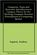Categories, Types, and Structures (Andrea Asperti, et al)

It introduces Category Theory at a level appropriate for computer scientists and provides practical examples in the context of programming language design, familiarizes readers with categorical concepts through examples based on elementary mathematical notions

•Logic, Programming and Prolog, 2nd Edition (Ulf Nilsson, et al)

This book introduces major new developments in a continually evolving field and includes such topics as concurrency and equational and constraint logic programming.

•An Introduction to Logic Programming Through Prolog (J. Spivey)

This is one of the few texts that combines three essential theses in the study of logic programming: logic, programming, and implementation. The techniques are illustrated by practical examples to explain how logic programming can be implented efficiently.

•Inductive Logic Programming: Techniques and Applications

This book is an introduction to inductive logic programming (ILP), which aims at a formal framework as well as practical algorithms for inductively learning relational descriptions in the form of logic programs.

•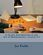A Gentle Introduction to the Art of Mathematics (Joseph E. Fields)

This book has chapters on Logic, Set theory, Relations and Cardinality interspersed with chapters on proofs - direct and indirect arguments, induction, combinatorial reasoning and "magic".

•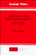Logics of Time and Computation (Robert Goldblatt)

This is a short but excellent introduction to modal, temporal, and dynamic logic, etc. It manages to cover, in highly readable style, the basic completeness, decidability, and expressability results in a variety of logics of the three kinds considered.

•Book of Proof, 3rd Edition (Richard H. Hammack)

This book is an introduction to the language and standard proof methods of mathematics. It lays a foundation for more theoretical courses such as topology, analysis and abstract algebra.

•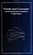Proofs and Concepts: The Fundamentals of Abstract Mathematics

This undergraduate textbook provides an introduction to proofs, logic, sets, functions, and other fundamental topics of abstract mathematics. It helps students transition from solving problems to proving theorems.

•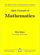Basic Concepts of Mathematics (Elias Zakon)

This book helps the student complete the transition from purely manipulative to rigorous mathematics, with topics that cover basic set theory, fields (with emphasis on the real numbers), a review of the geometry of three dimensions, and properties of linear spaces.

•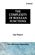The Complexity of Boolean Functions (Ingo Wegener)

Initially deals with the wee-known computation models, and goes on to special types of circuits, parallel computers, and branching programs.

•Normalization, Cut-Elimination, and the Theory of Proofs

This book is a concluding discussion focuses on the relationship between proofs and formal derivations, and the role proofs may play as part of a general theory of evidence.

•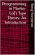Programming in Martin-Lof's Type Theory: An Introduction

This book focuses on the type theory developed by Per Martin-Lof. It contains a thorough introduction to the Martin-Lof's Type Theory, with information on polymorphic sets, subsets, monomorphic sets, and a full set of helpful examples.

•Logical Reasoning (Bradley H. Dowden)

The goal of this book is to improve your logical-reasoning skills. Your most important critical thinking skill is your skill at making judgments-not snap judgments that occur in the blink of an eye, but those that require careful reasoning.

•Foundations of Fuzzy Logic and Semantic Web Languages

This book provides a rigorous and succinct account of the mathematical methods and tools used for representing and reasoning with fuzzy information within Semantic Web languages. The book focuses on the three main streams of Semantic Web languages.

•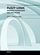Fuzzy Logic - Emerging Technologies and Applications (E. P. Dadios)

This book shows various applications of Fuzzy Logic in the field of Bioinformatics, Health, Security, Communications, Transportations, Financial Management, Energy and Environment Systems.

•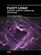Fuzzy Logic - Controls, Concepts, Theories and Applications

This book introduces new concepts and theories of Fuzzy Logic Control for the application and development of robotics and intelligent machines - how it will play a significant role in the development of intelligent robotic, machine, and mechatronics systems.

•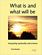What is and What will be: Integrating Spirituality and Science

This book integrates science with values and meaning by making the simplest possible assumption about the connection between physical structure and conscious experience. This follows from Gödel's Incompleteness Theorem and the assumptions of this book.

Book Categories
 :All CategoriesTop Free BooksRecent BooksMiscellaneous BooksComputer EngineeringComputer LanguagesComputer ScienceData Science/DatabasesJava and Java EE (J2EE)Linux and UnixMathematicsMicrosoft and .NETMobile ComputingNetworking and CommunicationsSoftware EngineeringSpecial TopicsWeb Programming
Other Categories